Looking for revision notes that are specific to the exam board you are studying? If so, click the links below to view our condensed, easy-to-understand revision notes for each exam board, practice exam question booklets, mindmap visual aids, interactive quizzes, PowerPoint presentations and a library of past papers directly from the exam boards.

# Oxidation Numbers, Redox and Half Equations

Chemical reactions are primarily the transfer of electrons. To understand the nature of this transfer and to identify the number of electrons taking part in this rearrangement, we need to understand the concept of oxidation states (number). Oxidation number of an atom is defined as “the charge that atom would have if the compound was composed of ions”. The definition for coordination compounds is a more complicated one according to IUPAC: “Of a central atom in a coordination entity, the charge it would bear if all the ligands were removed along with the electron pairs that were shared with the central atom. It is represented by a Roman numeral”. The actual distribution of electrons may or may not be exactly over a given atom in a molecule, but the concept of oxidation number helps us in simplifying the understanding of the complex reactions. Additionally, the oxidation number helps in exact systematic nomenclature of a compound.

Principles for finding oxidation states

1. An excess of electron is represented by the sign (-) while deficiency of electron is represented as (+)
2. Greater (+) sign indicates “higher oxidation state” while “lower oxidation state” is denoted by lesser (+)
3. Oxidation number of all the atoms in an element is zero
4. Total algebraic sum of the oxidation numbers of all the atoms in a neural compound must be zero
5. Total algebraic sum of the oxidation numbers of all the atoms in a ionic species is equal to the net charge
6. Oxidation number of a monoatomic ion is equal to its charge
7. Oxidation state of hydrogen in H+ is +1 but when it exists as a hydride ion H-, the oxidation state is -1
8. Usual oxidation state for oxygen is -2; exceptions contain O-O bonds like in O2, O3, H2O2, and O2-, O22-ion

The concept can be further clarified with the help of following examples:

Oxidation number of S in S8.

Since it is a pure element, the oxidation number of S = 0.

Oxidation number of Oxygen in O2, O2-, O22- and O2-

O2 is the elemental form and hence, the oxidation state of Oxygen = 0.

O2- is a monoatomic ion and hence, the oxidation state of Oxygen = the net charge = -2.

O22- is the peroxide ion and in this case, two negative charges are taken as distributed equally over the two O atoms and hence each one has an oxidation state = -1.

O2- is the superoxide radical and in this case, each of the O atoms have an oxidation state = -1/2.

Oxidation state for each element in H3PO4.

H3PO4 is constituted of three H+ ions and one PO43-. The obvious oxidation state of H is +1. The anion PO43- is a polyatomic ion in which the sum of charges of the P and four O must equal -3. Generally, O has the oxidation state of -2, which leaves P with oxidation state = -3 – [4(-2)] = -3 + 8 = +5.

Application of the concept of oxidation numbers is widespread in the balancing of “Redox” reactions. A redox reaction is the abbreviated form of oxidation-reduction reaction – a type of reaction involving a transfer of electrons between species so that these are oxidized and reduced at the expense of each other. For simplification, these two types of reactions are classified as:

(i) Reduction – gain of electrons and the lowering of oxidation number

(ii) Oxidation – loss of electrons and increase in oxidation number

The task of balancing the redox reactions involves the identification of “half reactions” – a name for representing the reduction and oxidation reactions separately. This method is also known as the ion-electron method.

Let’s look at an example for this. The reaction Zn(s) + Cu2+(aq) ® Cu(s) + Zn2+(aq) is a redox reaction in which Zn is oxidized while Cu is reduced. These are the two half reactions represented as:

Zn ® Zn2+(aq) + 2 e-
Cu ® Cu2+(aq) + 2 e-

A general guideline can be followed for writing and balancing half-reactions and overall redox process

• Step 1. Writing the skeleton equation for the whole redox reaction
• Step 2. Separation of the redox reaction into the appropriate half-reactions which further involves
• a) Assignment of oxidation numbers for all the atoms
• b) identification of all redox couples in the whole reaction and combining these redox couples into two appropriate half-reactions
• Step 3. Balancing the number of each type of atoms in both half reactions which further involves
• a) Balancing the number of every atom except H and O
• b) Balancing the number of O atoms with H2O
• c) Balancing the number of H atoms with H+
• d) For reaction in basic medium, adding one OH- for every H+ to both sides of the equation
• Step 4. Balancing the net charge by adding as many electrons
• Step 5: Making the loss and gain of electrons equivalent, in the half-reactions
• Step 6: Adding the half-reactions
• Step 7: Simplifying the overall redox equation
• Finally, a check needs to be done to confirm the balance of charges and elements

An example is given below to illustrate the balancing a redox reaction by “half-reaction” method.

Let’s look at the reaction: MnO4- + SO32- = MnO2 + SO42-

Reaction in basic medium

Step 1.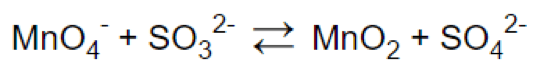Step 2.

(a)(b) & (c)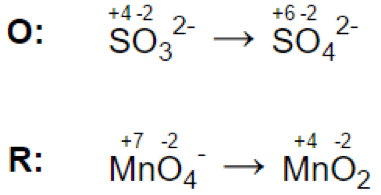Step 3.

(a)(b)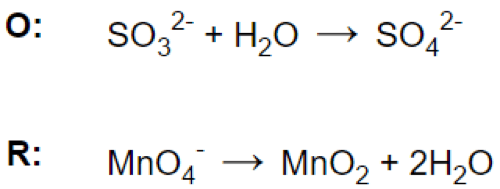(c)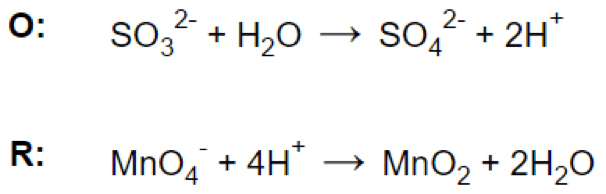(d)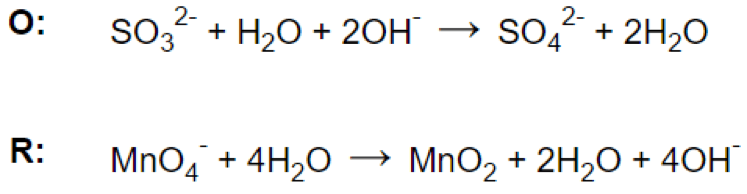Step 4.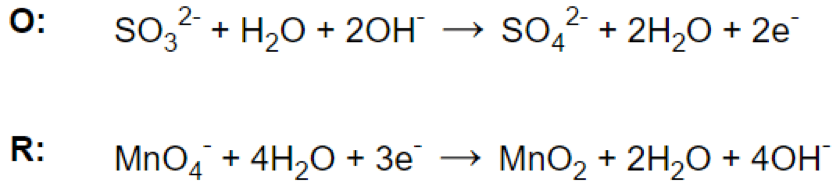Step 5.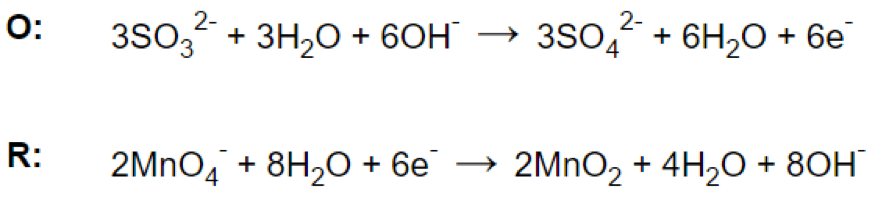Step 6.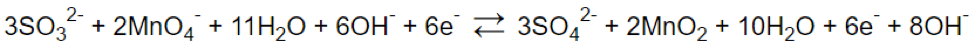Step 7.Reaction in acidic medium

Step 1.Step 2.

(a)(b) & (c)Step 3.

(a)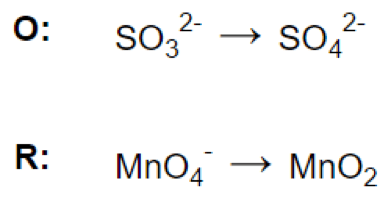(b)(c)Step 4.Step 5.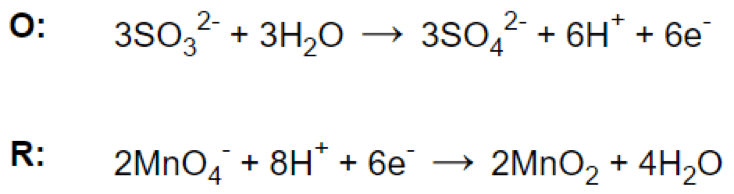Step 6.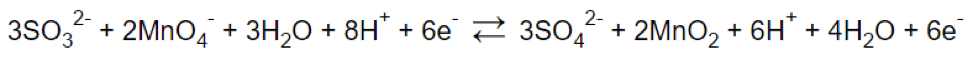Step 7.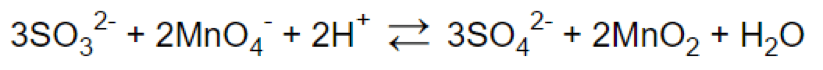References: